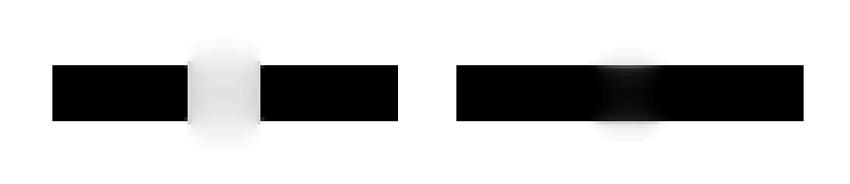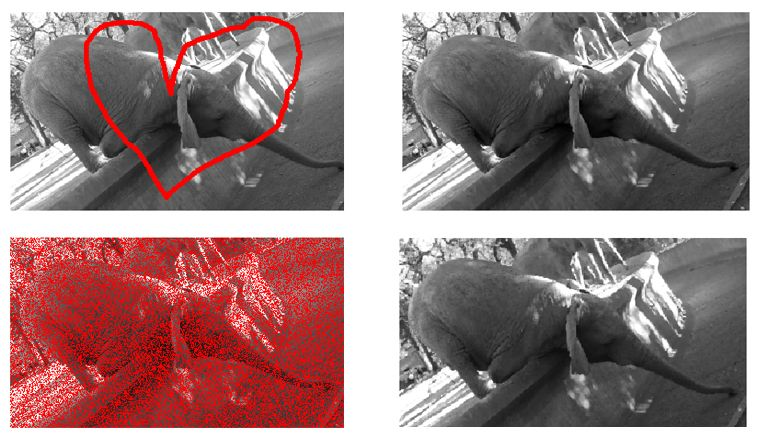# Applying Modern PDE Techniques to Digital Image Restoration

By Carola-Bibiane Schönlieb, University of Cambridge

Inpainting, or image interpolation, is a process used to reconstruct missing parts of images. Artists have long used manual inpainting to restore damaged paintings. Today, mathematicians apply partial differential equations (PDEs) to automate image interpolation. The PDEs operate in much the same way that trained restorers do: They propagate information from the structure around a hole into the hole to fill it in [1,2,3].

While artists can work directly on a painting, a PDE requires a mathematical representation of the subject matter, such as a digital image. A digital image is essentially a 2D matrix of integers, with each integer representing the color or grayscale value of an individual pixel. Holes in the image are represented by unknown values in the matrix. PDE-inpainting fills in these missing values based on the values of nearby pixels.

One of my first explorations of the use of PDEs for inpainting focused on the restoration of 14th-century frescoes. I have found that high-order, nonlinear PDEs enable a wide range of other applications for inpainting, in fields as diverse as medicine, astronomy, and cartography.

For me, MATLAB® is a natural choice for inpainting with PDEs. MATLAB enables me to rapidly develop and test preliminary algorithms. The MATLAB operations in my scripts have a virtually one-to-one relationship with the steps in the algorithms I develop. This level of correspondence is all but impossible to achieve using a lower-level programming language. I can easily visualize the results in many different formats using graphs and plots, a type of graphical analysis that, with a lower-level language, often requires a second tool.

## Simple Inpainting: Fourier's Heat Equation

Fourier's heat equation describes how temperature in a material changes. Given this PDE, knowledge of the thermal conductivity of the material, and knowledge of the initial temperature conditions u(t=0), it is possible to calculate the temperature at any point in the material for any given time by

$u_t = \Delta u$

The heat equation is also known as the diffusion equation because it describes how a value (in this case, temperature) diffuses across an area over a time interval.

With grayscale values replacing temperature, a slightly modified version of this equation can be used for basic inpainting. I implemented a simple MATLAB program that uses this technique. This program can restore an image with holes cut in it by propagating (or diffusing) the grayscale values surrounding each hole toward the hole's center (Figure 1).

This approach works well only when the areas surrounding each hole are homogenous. When the hole spans a sharp edge, the edge pixels are diffused, causing the edge to be lost in the restored section (Figure 2).

## Handling Nonlinearity: The Perona-Malik Equation

Because the heat equation possesses only smooth solutions, it cannot preserve discontinuous image features such as edges. In particular, it cannot propagate edges across large holes because it relies on linear interpolation. In Figure 2, these edges (the dark parallel lines on either side of the hole) are not propagated into the hole by the inpainting process.

To address this shortcoming, I created a second MATLAB program that uses nonlinear diffusion. This program uses total variation flow , a technique in which the diffusivity constant depends upon the size of the image gradient, reducing the amount of diffusion that occurs near edges.

Starting with the initial guess $$u(t=0)$$ for the image inside the hole, an inpainted image $$u(t=T)$$ is constructed by evolving

$u_t = \mathrm{div}\left(\frac{\nabla u}{|\nabla u|}\right)$

This program, which uses a form of the Perona-Malik equation , preserves edges in an image and to some extent propagates them into missing regions (Figure 3).

Although total variation flow is a clear improvement over the heat equation approach, it still has disadvantages. Because the Perona-Malik equation is a second-order PDE, it does not perform well on edges that span large gaps or holes in an image (Figure 4). The equation cannot incorporate information about the direction in which to continue an edge. As a result, the edge fades away into the hole.

## Factoring in Gradients: Fourth-Order Total Variation Equation

Higher-order PDEs address the deficiencies of the total variation flow approach. With a MATLAB program that uses fourth-order PDEs, we can take two boundary conditions into account instead of just one. Specifically, instead of factoring in only the grayscale value of the pixels near a hole, the program also factors in the gradient, which enables it to model the direction in which edges should be propagated into the hole.

Material science researchers often use the Cahn-Hilliard equation to model phase separation, a process in which the constituents of a fluid mixture spontaneously separate, coarsening the phases. The Cahn-Hilliard equation has been very successfully applied to the inpainting of binary structures with large gaps . A generalization of this equation for grayvalue images  can be shown to be equivalent to a fourth-order version of total variation flow:

$u_t = - \Delta \left(\mathrm{div}\left(\frac{\nabla u}{|\nabla u|}\right)\right)$

I implemented this fourth-order total variation equation in MATLAB to reconstruct the edges even within large holes (Figure 5). The complete algorithm comprises about 200 lines of MATLAB code, and it uses only basic matrix operations and calls to fft() to perform fast Fourier transforms.Figure 5. Left: The total variation flow reconstruction of Figure 4. Right: The same image reconstructed using the fourth-order total variation flow (generalized Cahn-Hilliard equation).

Figure 6 shows more elaborate examples using this inpainting equation. Although the number of missing pixels in the first example is only one tenth of the number of missing pixels in the second example, the correct inpainting is much harder to achieve for the former. This is because the diameter of the inpainting domain (the width of the holes) is the crucial factor rather than their area. Especially in the more difficult situation of the first example in Figure 6, fourth-order and nonlinear PDEs are of utmost importance.Figure 6. Top and bottom left: Images with different kinds of holes. Top and bottom right: Corresponding fourth-order total variation reconstructions.

The solution to the fourth-order total variation equation for inpainting is both space-dependent and time-dependent. Using an explicit method to solve this fourth-order PDE would be computationally prohibitive: Each time step involves complex matrix multiplication operations, and it would take thousands of time steps to ensure that the algorithm converged. The MATLAB algorithm that I developed uses a semi-implicit method for time discretization that linearizes the problem at every time step and then solves a linear system of equations . For space discretization, the algorithm uses finite difference methods. These methods work well because images are essentially fixed, equidistant grids of pixels.

## Applications of PDE-Based Inpainting

My main work with the Cahn-Hilliard inpainting began on a project to restore some 14th-century Viennese frescoes that were rediscovered in 1979 (Figure 7).

I recently worked on a project to map a network of roads from satellite images. Numerous objects, including cars and trees, obscured the roads in the images. Using MATLAB and Cahn-Hilliard inpainting, my colleagues and I developed an algorithm that automatically removed these obstructions from the satellite images. French astronomers are using a similar technique to remove stars in the Milky Way from astronomical images so as to obtain a clearer picture of the galaxies beyond. PDE-based inpainting algorithms are also applicable in the medical field to reconstruct images from highly undersampled MRI measurements.

## New Trends in Inpainting

Not all inpainting methods rely on PDEs. For example, exemplar-based inpainting uses a copy-and-paste algorithm to fill in missing areas of an image. If an image has a hole where a missing shirt button should be, for example, an algorithm that uses exemplar-based inpainting could insert the button by copying one from another location in the image; a PDE-based algorithm could not.

Exemplar-based inpainting is a nonlocal technique–it uses information from the whole image, not just from the area surrounding the image. While exemplar-based inpainting provides impressive results in the restoration of textiles and repetitive structures , its ability to reconstruct structures without a given example is limited. For this reason, a synthesis of local (PDE) and non-local (exemplar-based) inpainting techniques is desirable, and current research is focusing on this approach.

Carola-Bibiane Schönlieb is a lecturer in Applied and Computational Mathematics in the Department of Applied Mathematics and Theoretical Physics at Cambridge University, and a Fellow of Jesus College, Cambridge. She uses the algorithms that she developed in MATLAB in her teaching, including the course Modern PDE Techniques for Image Inpainting. Dr. Schönlieb holds an M.A. in mathematics from the University of Salzburg and a Ph.D. in mathematics from the University of Cambridge.

Published 2012 - 91992v00

### References

1. M. Bertalmio, G. Sapiro, V. Caselles, and C. Ballester, "Image Inpainting, Siggraph 2000," Computer Graphics Proceedings, pp.417-424 (2000).
2. T.F. Chan and J. Shen, "Variational Image Inpainting," Comm. Pure Applied Math, Vol. 58, pp. 579-619 (2005).
3. F. Bornemann and T. März, "Fast Image Inpainting Based on Coherence Transport," J. Math. Imaging Vis. 28, pp. 259-278 (2007).

4. P. Perona, and J. Malik, "Scale-space and Edge Detection Using Anisotropic Diffusion," IEEE Transactions on Pattern Analysis and Machine Intelligence, 12(7), pp.629-639 (1990).
5. A. Bertozzi, S. Esedoglu, and A. Gillette, "Inpainting of Binary Images Using the Cahn-Hilliard Equation," IEEE Trans. Image Proc. 16(1) pp. 285-291 (2007).
6. M. Burger, L. He, C.-B. Schönlieb, "Cahn-Hilliard inpainting and a generalization for grayvalue images," SIAM J. Imaging Sci. Volume 2, Issue 4, pp. 1129-1167 (2009).
7. C.-B. Schönlieb, A. Bertozzi, "Unconditionally stable schemes for higher order inpainting," Communications in Mathematical Sciences Volume 9, Issue 2, pp. 413-457 (2011).
8. V. Caselles, "Exemplar-Based Image Inpainting and Applications," ICIAM 2011, SIAM News, Volume 44, Number 10, December 2011.